## Do I Have Enough? - A Macro for Sample Size Determination in Simple and Multiple Logistic Regression

Started ‎04-15-2021 by
Modified ‎05-12-2021 by
Views 1,754
Paper 1158-2021

AUTHORS: Carl P. Wilson, Spectrum Health Office of Research and Education and Grand Valley State University

## Abstract

Binary logistic regression finds plenteous usage throughout many scientific disciplines. Despite its multitudinous applications, there lacks a universal method of determining the sample size for a binary logistic regression model. Some suggest using ‘magic number’ methods to estimate the sample size, but such methods do not include statistical power, effect size, or significance level into their approximations. To remedy this dilemma, this paper presents a SAS macro that automates the sample size determinations for both simple and multiple binary logistic regression models with continuous predictors. This macro—based on Hsieh’s (1998) formula—lacks the limitations from which the magic number method tolerates. Researchers will benefit from using this macro to determine the true sample sizes for their studies and to overcome the imbroglios of under and overestimating a sample size.

## Watch the Presentation

Watch Do I Have Enough? - A Macro for Sample Size Determination in Simple and Multiple Logistic Regression as presented by the author on the SAS Users YouTube Channel.

## Introduction

The application of binary logistic regression is prevalent throughout various scientific disciplines, such as biomedicine, social science, business, and genetics (Agresti, 2014). The ubiquity of binary logistic modeling compels the desideratum to obtain a proper sample size calculation. Generally speaking, with an insufficient sample size, a study may fail to detect even large treatment effects (Wang & Ji, 2020). Conversely, a study may allocate expensive resources (e.g. research time, personnel labor, overall cost) towards obtaining an excessively inflated sample size.

To further complicate things, there is an egregious amount of disagreement regarding the best method of sample size determination for logistic regression. One proposed solution, the EPV method (Events Per Variable), suggests having a specified minimum number of events/subjects per explanatory variable (Harrell, Lee, Matchar & Reichert, 1985). Both cox regression and logistic regression used this methodology with the generally advocated EPV value being ten (Peduzzi, et. al, 1996), (Concato, et. al, 1995). Unfortunately, there is even discordance for the ideal EPV value, with some disputing it should be at least 20 (Austin & Steyerberg, 2017), and others suggesting the minimum should be 50 (Bujang, et. al, 2018). There is also speculation regarding whether the EPV method is even valid (Smeden et al., 2016). Further inspecting validity, these “Magic Number” methods do not take into consideration an effect size, significance level, or power threshold for their sample size calculations, and are therefore less apt than methods that do (Courvoisier, et. al, 2011). In order to rectify these issues, this macro uses an approach by Hsieh (1998).

# BACKGROUND

Hsieh’s method of sample size determination is a formulaic approach predicated on a binary logistic regression model being generalized by a two-sample framework. In a simple logistic regression relating a predictor X1 to a binary response Y, the model is as follows: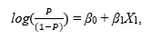In such a scenario, we are interested in whether or not the predictor X1 is related to the binary response variable, and we verify this by testing the null hypothesis H0: β1= 0, against the alternative hypothesis H1: β1 ≠ 0. When X1 is a continuous variable with a normal distribution, the log odds value β1 = 0, if and only if, the group means (assuming homogeneity of variances) is equal between the two response categories; therefore, we can derive a sample size formula from a two-sample test (see Hsieh (1998) for full derivation and theory). As suggested by Whittemore (1980), the sample size approximation can be further improved for small response probabilities via multiplying by a correction factor of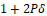, yielding a sample size formula of: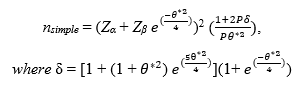Zα and Zβ are the upper quantiles for the significance and power thresholds respectively, P =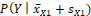, the probability of the response at one standard deviation above the mean of the predictor X1, and θ* = log(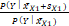), the overall effect size derived as the log odds ratio of the probability of the response at one standard deviation above the mean of X1, relative to the probability of the response at the mean of X1. As the probability of the response at the mean of X1 approaches 0.50, and as |θ*| increases, the smaller the necessary sample size to achieve the desired power will be (see Agresti (2014) for more information).

Whittemore (1981) and Hsieh (1998) demonstrated that this formula can also be used to approximate the sample size for a multiple logistic regression model with n continuous predictors, through inflating the aforementioned simple logistic regression sample size via multiplying by 1/R2, where R2 is the proportion of the variance of X1 (the original predictor from the univariable model) explained by the regression with the new quantitative predictors in the multiple variable model, X2, …, Xn.. This yields a sample size formula of: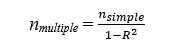## EXPLANATION OF THE MACRO FUNCTIONALITY AND CODE

This macro has the capacity to automatically calculate the effect size for the user, a feature that most sample size determination software’s lack. However, this is at the expense of requiring a sample dataset to derive the calculations from. This macro will be useful for researchers conducting small-scale pilot studies, as they can determine an effect size and required sample size to achieve their desired power and significance levels for their full-scale research projects.

As aforementioned, the macro is derived from Hsieh and Whittemore’s formulas and is flexible in that it can automate the determination of the sample size both for simple and multiple binary logistic regressions. The macro has 6 parameters, and are outlined as follows:

• dataset – The name of the dataset.
• response – The binary response variable.
• x – The singular continuous predictor for the simple logistic regression case, or the main predictor of interest for the multiple logistic regression case.
• other_x – The other continuous predictors for the multiple logistic regression case; to utilize only a simple logistic regression, set this parameter equal to 0.
• alpha – The specified significance level.
• beta – The specified beta level to calculate power (power = 1 - β)

## EXAMPLE OF MACRO CODE USE

SASHELP library contains the dataset HEART, which we will use to illustrate the functionality of this macro. The HEART dataset contains data from the Framingham heart study (see framinghamheartstudy.org/fhs-about/ for more information), and with it, we can build simple and multiple logistic regressions to predict the binary variable status (Dead or Alive) based on continuous predictors. To parallel how this macro may be used in a research setting, a random sample of size 100 will be taken via PROC SURVEYSELECT. The random sample is comparable to how a researcher would use this macro with a small-scale pilot dataset. From this pilot dataset, they use the macro to determine the observed effect size, and with this value, the macro calculates the necessary sample size to reach the power and significance levels desired for their full-scale study. The source code used to create the random sample is given below:

``````proc surveyselect data=sashelp.heart out=sample
sampsize=100 seed=12345;
run;``````

### Simple Logistic Regression

If we are only interested in predicting status based one main predictor of interest (i.e. systolic blood pressure), and we want a significance level of α = 0.05, and a power of 0.8, the macro code is as follows:

``````%mlog_n(dataset = sample,
response = Status,
x = systolic,
other_x = 0,
alpha = .05,
beta =  .2);
run;``````

For this example, the dataset parameter is sample (the random sample of 100 observations from the HEART dataset), the response is status, the predictor x is systolic, the parameter for the other predictors other_x will be set to zero as we are only interested in one predictor, systolic. Our specified alpha threshold is 0.05, and our beta level is 0.2 (i.e. 1 - .2 = .8 power).

The macro outputs summary values of the input parameters, such as the significance level, the power threshold, the respective upper quantiles of the two aforementioned values, the probability of the response at the mean level of the predictor variable X, the probability of the response at 1 standard deviation above the mean of the predictor X, the overall log-odds (effect size), and the value of Whittemore’s (1980) correction factor. Subsequent to the summary values, the macro outputs the estimated sample size based on the input parameters via Hsieh’s (1998) formula (Output 1).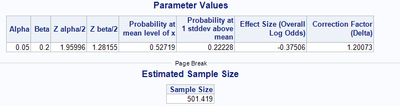Output 1. Output for Simple Logistic Regression

### Multiple Logistic Regression

We can also create a multiple binary logistic regression model by including more predictors (e.g., systolic, diastolic, weight, height, and cholesterol) to predict status. Using the same significance and power as the previous example, the macro code is as follows:

``````%mlog_n(dataset = sample,
response = Status,
x = systolic,
other_x = diastolic height weight cholesterol,
alpha = .05,
beta =  .2);
run;``````

For this example, the dataset parameter is still the random sample, the response remains as status; the x parameter remains as systolic for being our main predictor of interest; the other_x parameters are now diastolic, height, weight; and cholesterol instead of 0, and lastly our alpha and beta thresholds are still 0.05 and 0.2, respectively.

The macro outputs the same summary values of the input parameters and sample size that the simple logistic regression version does, as well as the sample size estimation for the multiple logistic regression (Output 2).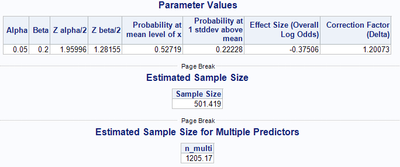Output 2. Output for Simple Logistic Regression

## Conclusion

With the importance of a proper sample size for conducting research, this macro is beneficial in that it streamlines the process of sample size determination for simple and multiple binary logistic regression models. It provides novel usefulness in that the macro can automate the calculation of the effect size θ* and the sample size n from a smaller representative sample, allowing the researcher to know how much data they will need to collect or pull from databases/registries in order to achieve their desired power and significance levels.

In both of these scenarios, the macro circumvents the issue of failing to detect effects attributed to having too small of a sample size—a complication that can arise from using “magic number” methods like the Events Per Variable method, which do not consider statistical power, significance, or an effect size. This macro also saves resources such as cost, labor, and research time that would be drained from excessive data collection to achieve a larger than necessary sample size.

## References

Agresti, A. (2014). Categorical Data Analysis. Hoboken: Wiley.

Austin, P. C., & Steyerberg, E. W. 2017. “Events per variable (EPV) and the relative performance of different strategies for estimating the out-of-sample validity of logistic regression models.” Statistical methods in medical research26(2), 796–808. https://doi.org/10.1177/0962280214558972

Bujang, M. A., Sa'at, N., Sidik, T., & Joo, L. C. 2018. “Sample Size Guidelines for Logistic Regression from Observational Studies with Large Population: Emphasis on the Accuracy Between Statistics and Parameters Based on Real Life Clinical Data.” The Malaysian journal of medical sciences : MJMS25(4), 122–130. https://doi.org/10.21315/mjms2018.25.4.12

Concato, J., Peduzzi, P., Holford, T. R., & Feinstein, A. R. 1995. “Importance of events per independent variable in proportional hazards analysis. I. Background, goals, and general strategy.” Journal of clinical epidemiology48(12), 1495–1501. https://doi.org/10.1016/0895-4356(95)00510-2

Courvoisier DS, Combescure C, Agoritsas T, Gayet-Ageron A, & Perneger TV. 2011. “Performance of logistic regression modeling: beyond the number of events per variable, the role of data structure.” J Clin Epidemiol.

Harrell FE Jr, Lee KL, Matchar DB, Reichert TA. 1985. “Regression models for prognostic prediction: advantages, problems, and suggested solutions.” Cancer Treat Rep.

Hsieh, F. Y., Bloch, D. A., & Larsen, M. D. 1998. “A simple method of sample size calculation for linear and logistic regression.” Statistics in Medicine, 17(14), 1623-1634. http://dx.doi.org/10.1002/(SICI)1097-0258(19980730)17:14<1623::AID-SIM871>3.0.CO;2-S

Peduzzi P, Concato J, Kemper E, Holford TR, Feinstein AR. 1996. “A simulation study of the number of events per variable in logistic regression analysis.” J Clin Epidemiol.

Smeden, M. V., Groot, J. A., Moons, K. G., Collins, G. S., Altman, D. G., Eijkemans, M. J., & Reitsma, J. B. 2016. “No rationale for 1 variable per 10 events criterion for binary logistic regression analysis.” BMC Medical Research Methodology, 16(1). https://doi.org/10.1186/s12874-016-0267-3

Wang, X., & Ji, X. 2020. “Sample Size Estimation in Clinical Research From Randomized Controlled Trials to Observational Studies.” An Overview Of Study Design And Statistical Considerations, 158(1). https://doi.org/10.1007/s11707-018-0727-7

Whittemore, A. 1981. “Sample size for logistic regression with small response probability.”

Journal of the American Statistical Association.

## Acknowledgments

I would like to acknowledge the entire Scholarly Activity and Scientific Support team at Spectrum Health for their feedback on this paper. A special thanks to Nicholas Andersen, PhD, for his guidance in the development of this paper, and also to Jessica Parker for her continued mentoring throughout the entirety of my SAS Global Forum experience.

(PDF) A Simple Method of Sample Size Calculation for Linear and Logistic Regression (researchgate.ne...

## Contact Information

Your comments and questions are valued and encouraged. Contact the author at:

Carl P. Wilson

Spectrum Health Office of Research and Education

Grand Valley State University

wilsocar@mail.gvsu.edu

SAS and all other SAS Institute Inc. product or service names are registered trademarks or trademarks of SAS Institute Inc. in the USA and other countries. ® indicates USA registration.

Other brand and product names are trademarks of their respective companies.

Version history
Last update:
‎05-12-2021 11:01 AM
Updated by:Contributors
Article Labels
Article Tags